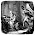## sábado, 13 de junio de 2020

### Geometric Proof of the Sum Angle Formula (Sine, Cosine)

I wouldn't say the simpler one but at least $\sin(α+β)$ can be obtained almost without words.

$\frac{\cos{\alpha}}{\cos{\beta}}-\frac{\sin{\beta}(\sin{\alpha}\cos{\beta}+\cos{\alpha}\sin{\beta})}{\cos{\beta}}=\frac{\cos{\alpha}-\sin^2{\beta}\cos{\alpha}-\sin{\beta}\sin{\alpha}\cos{\beta}}{\cos{\beta}}=\frac{\cos{\alpha}(1-\sin^2{\beta})-\sin{\beta}\sin{\alpha}\cos{\beta}}{\cos{\beta}}=$

$\frac{\cos{\alpha}\cos^2{\beta}-\sin{\beta}\sin{\alpha}\cos{\beta}}{\cos{\beta}}=\cos{\alpha}\cos{\beta}-\sin{\beta}\sin{\alpha}=\cos{(\alpha+\beta)}.$

#### 3 comentarios:

1.1.2.